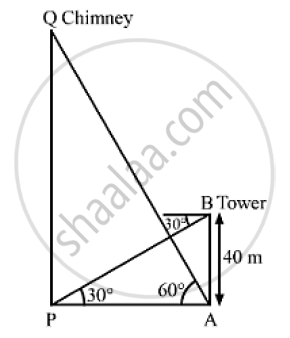# He Angle of Elevation of the Top of a Chimney Form the Foot of a Tower is 60° and the Angle of Depression of the Foot of the Chimney from the Top of the Tower is 30° Find the Height of the Chimney. - Mathematics

The angle of elevation of the top of a chimney form the foot of a tower is 60° and the angle of depression of the foot of the chimney from the top of the tower is 30° . If the height of the tower is 40 meters. Find the height of the chimney.

The angle of elevation of the top of a chimney from the foot of a tower is 60° and the angle of depression of the foot of the chimney from the top of the tower is 30°. If the height of the tower is 40 m, find the height of the chimney. According to pollution control norms, the minimum height of a smoke emitting chimney should be 100 m. State if the height of the above mentioned chimney meets the pollution norms. What value is discussed in this question?

#### SolutionLet PQ be the chimney and AB be the tower.
We have,
AB = 40m,∠APB = 30°  and ∠PAQ = 60°
In ΔABP,

tan 30° = (AB)/(AP)

⇒ 1/ sqrt(3) = 40/ (AP)

⇒ AP = 40 sqrt(3 ) m

Now, in Δ APQ ,

 tan 60° = (OQ)/(AP)

⇒  sqrt(3) = (PQ) / (40 sqrt(3))

∴ PQ = 120 m

So, the height of the chimney is 120 m.
Hence, the height of the chimney meets the pollution norms.
In this question, management of air pollution has been shown

Concept: Heights and Distances
Is there an error in this question or solution?
Chapter 14: Height and Distance - Exercises

#### APPEARS IN

RS Aggarwal Secondary School Class 10 Maths
Chapter 14 Height and Distance
Exercises | Q 18

Share